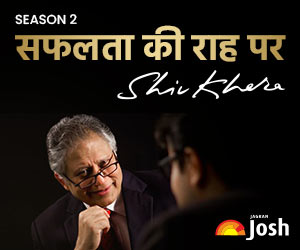# SCRA Exam Paper on Mathematics - 2011

Find here 2011 SCRA Question Paper on Mathematics.Solve the SCRA Exam Question Paper of the previous year. Prepare better.

Mathematics

2. In an examination of a certain class, at least 70% of the students failed in Physics, at least 72% failed in Chemistry, at least 80% failed in Mathematics and at least 85% failed in English. How many at lest must have failed in all the four subjects ?

(a) 5%

(b) 7%

(c) 15%

(d) Cannot be determined due to insufficient data

3. If a≠0 and the line 4bx+3cy+2d=0 passes throught the points of intersection of the paraboals y2=4ax and x2=4ay, then which one of the following is correct ?

(a) d2+(3b-4c)2=0

(b) d2+(3b=4c)2=0

(c) d2+(4b+3C)2=a2

(d) d2+(4b+3c)2=0

5. Which one of the following represents the curve in the xy-plane passing through the point (2,2) and having at each of its points the slope equal to -x-2 ?(a) y=x=1.5

(b) y=1.5x

(c) xy=1.5

(d) xy=1.5x+1

7. What is the range of the function f (x)=x-[x] where [x] is the greatest integer function ?

(a) [0,1)

(b) (0, 1]

(c) (0,1)

(d) [0,1]

8. If the lines 3x-4y+4=0 and 6x-8y-7=0 are tangents to the circle, then what is the radius of the circle ?

(a) 3/4

(b) 4/3

(c) 3/2

(d) 3